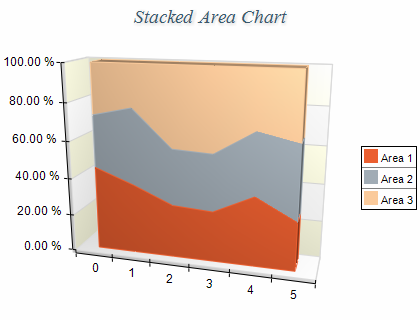﻿ Stacked Percent Area
Nevron .NET Vision Documentation
Stacked Percent Area

Stacked Percent Area charts represent the relative contribution of data series to the total for each category. The series are displayed as sequences of straight line segments and the area below each line is filled. Each area series is stacked on top of the previous one. The height of the uppermost line is always the full height of the chart. The Y scale range is always from 0 to 1. The following figure displays a Stacked Percent Area chart:Figure 1.

Creating the area series

Stacked Percent Area charts are displayed with several NAreaSeries objects. The MultiAreaMode property of the first area series must be set to MultiAreaMode.Series. The MultiAreaMode property of the subsequent area series must be set to MultiAreaMode.StackedPercent. The following example demonstrates how to create a Stacked Percent Area chart with three series:

C#
Copy Code
```// obtain a reference to the Cartesian chart that is created by default
NCartesianChart chart = (NCartesianChart)chartControl.Charts;

area1.MultiAreaMode = MultiAreaMode.Series;
area2.MultiAreaMode = MultiAreaMode.StackedPercent;
area3.MultiAreaMode = MultiAreaMode.StackedPercent;
```
Visual Basic
Copy Code
```' obtain a reference to the Cartesian chart that is created by default
Dim chart As NCartesianChart = chartControl.Charts(0)

Dim area1 As NAreaSeries = chart.Series.Add(SeriesType.Area)
Dim area2 As NAreaSeries = chart.Series.Add(SeriesType.Area)
Dim area3 As NAreaSeries = chart.Series.Add(SeriesType.Area)

area1.MultiAreaMode = MultiAreaMode.Series
area2.MultiAreaMode = MultiAreaMode.StackedPercent
area3.MultiAreaMode = MultiAreaMode.StackedPercent
```
Stacked Percent Scaling

When an area series is displayed in stacked percent mode the min value of the series is always 0 and the max value is always 1. In order to display the texts on the PrimaryY axis as percents you have to use the following code:

C#
Copy Code
```NStandardScaleConfigurator sc = (NStandardScaleConfigurator)chart.Axis(StandardAxis.PrimaryY).ScaleConfigurator;
sc.LabelValueFormatter = new NNumericValueFormatter(NumericValueFormat.Percentage);
```
Visual Basic
Copy Code
```Dim sc As NStandardScaleConfigurator = chart.Axis(StandardAxis.PrimaryY).ScaleConfigurator
sc.LabelValueFormatter = New NNumericValueFormatter(NumericValueFormat.Percentage)
```
Stacks with negative values
Stacked percent area charts do not support negative values, which means that all values are internally converted to their absolute value.
Formatting Commands

The following formatting commands inherited from the NSeries class have different meaning when an area series is stacked:

<total> - displays the absolute sum of the values in the current stack.
<cumulative> - displays the sum of the values up to the current stack value.
<percent> - displays the percent contribution of the value to the total pile sum.

Related Examples
Windows forms: Chart Gallery\Area\Stacked Area